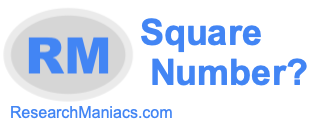Is 1 a square number?Here we will answer the question: "Is 1 a square number?" You could also ask "Is 1 a perfect square?" Square number and perfect square mean the same thing.

Anyway, for 1 to be a square number, 1 must be the product of an integer multiplied by itself. In other words, the square root of 1 must equal a whole number (integer).

The square root of 1 is 1 which is an integer. Thus, 1 is a square number.

The answer to the question: "Is 1 a square number?" is Yes.
The answer to the question: "Is 1 a perfect square?" is also Yes.

Square Number?
Enter another number to see if it is a square number:

Is 2 a square number?
Find out if the next number on our list is a perfect square.